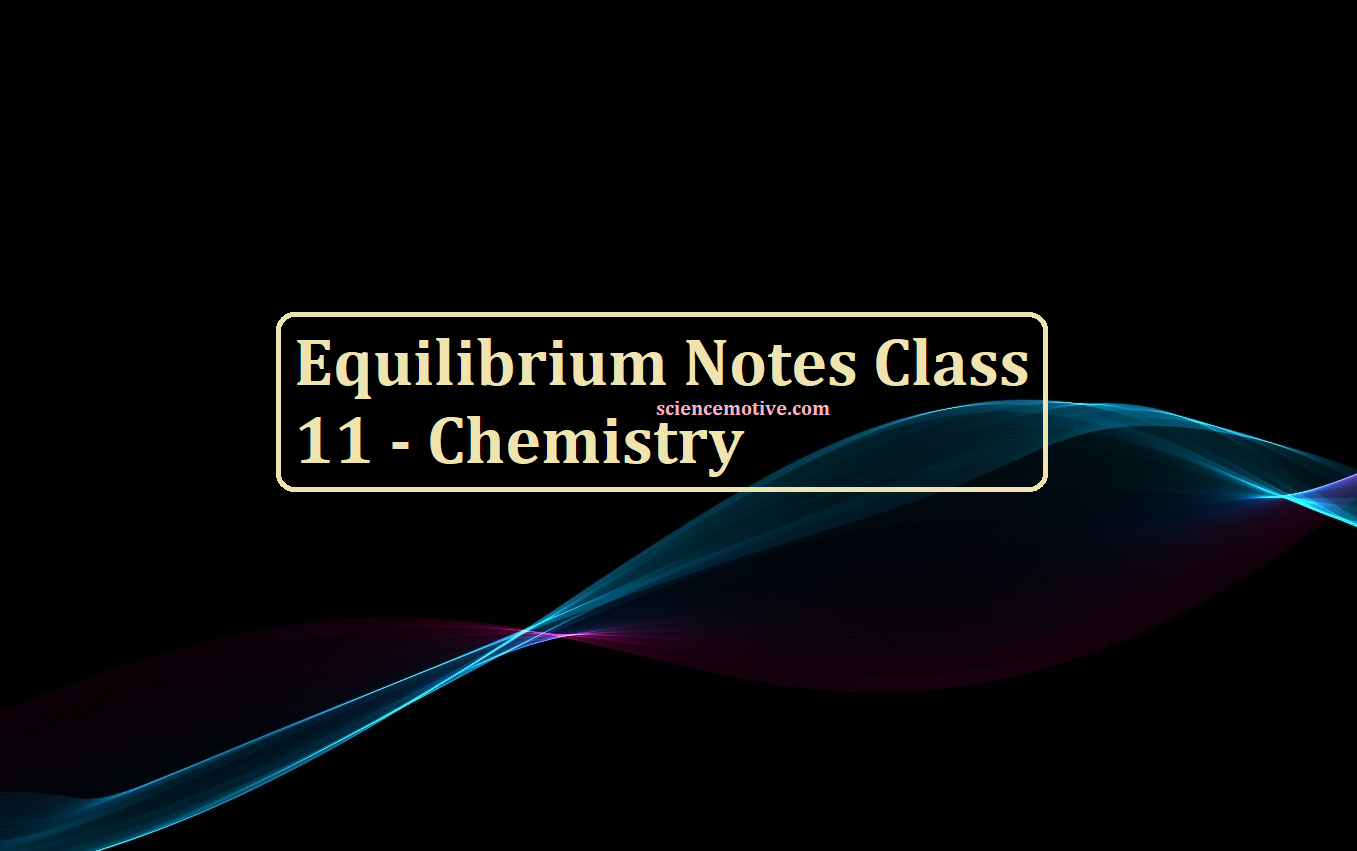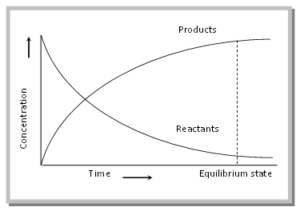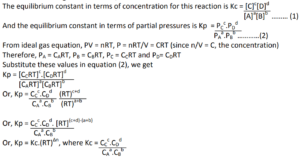# Equilibrium Notes Class 11 – Chemistry# Equilibrium Notes Class 11

Equilibrium Notes Class 11

An Equilibrium represents the state of a process in which the properties like temperature, pressure, the concentration of the system does not show any change with the passage of time

Equilibrium is the state at which the concentration of reactants and products do not change with time. i.e., concentrations of reactants and products become constant.
Types: Equilibrium in a system implies the existence of the following types of equilibria simultaneously,

(i) Physical equilibrium: It is the process that involves physical changes only, like an equilibrium between different states of substances at a particular temperature.
→ Solid ⇌ Liquid: ice water at 0°C rates of melting = rate of freezing
→ Liquid ⇌ Gas (vapour): Water (liquid) water (vapours) at 100 °C rate of evaporation = rate of condensation
→ Solid ⇌ Gas (vapour): CO2 (solid) CO2 (vapours) rate of sublimation = rate of condensation
→ Solid ⇌ Saturated solution of solids in the liquid rate of dissolution = rate of precipitation
→ Gas ⇌ Saturated solution of the gas in a liquid is always exothermic and spontaneous.

(ii) Chemical Equilibrium: Equilibrium associated with chemical reactions is called chemical equilibrium. At equilibrium, the concentrations of reactants and products are constant.
Consider a hypothetical reaction, A + B ⇌ C + D
As the reaction proceeds, the concentration of the reactants decreases, and that of the products increases. After some time, the two reactions occur at the same rates, and an equilibrium state is reached. This can be illustrated by the following graph.After the equilibrium is attained, the concentration of the reactants and products becomes constant. At equilibrium, The rate of forwarding reaction (rf) = The rate of backward reaction (rb).
General characteristics of chemical equilibrium:
→ It is dynamic in nature.
→ The observable properties of the system become constant at equilibrium and remain unchanged thereafter.
→ The equilibrium can be approached from either direction.
→ The equilibrium can be attained only if the system is a closed one.
→ The free energy change at constant pressure and temperature is zero.
→ The addition of a catalyst does not change the equilibrium state, it only helps in attaining the equilibrium faster.
There are two types of chemical equilibrium:
→ Homogeneous equilibrium: The equilibrium reactions in which all the reactants and the products are in the same phase are called homogeneous equilibrium reactions.
Example: (i) C2H5OH (l) + CH3COOH (l) ⇌ CH3COOC2H5 (l) + H2O (l)
(ii) N2 (g) + 3 H2 (g) 2 NH3
(iii) 2 SO2 (g) + O2 (g) 2 SO3
→ Heterogeneous equilibrium: The equilibrium reactions in which the reactants and the products are present in different phases are called heterogeneous equilibrium reactions.
Example: (i) CaCO3 (s) ⇌ CaO (s) + CO2
(ii) 2 NaHCO3 ⇌ Na2CO3 (s) + CO2 (g) + H2O (g)
(iii) H2O (l) ⇌ H2O (g)

Equilibrium Notes Class 11

Reversible and Irreversible Reactions:
→ Reversible reaction: A reaction in which the reactants are formed back by the reaction of products with each other at the given conditions of the reaction. These reactions if carried out in a closed vessel do not go to completion. e.g.,
CH3COOH + C2H5OH CH3COOC2H5 + H2O
N2(g) + 3H2(g) 2NH3(g)
The process by which reactants are converted to products is called forward reaction and the process by which products recombine to form reactants is called backward reaction. After some time, the rate of forwarding reaction becomes equal to the rate of backward reaction and the reaction attains equilibrium. Thus, equilibrium is a state in which the rates of forwarding and backward reactions are equal.
Characteristics of reversible reactions
(a) These reactions can be started from either side,
(b) These reactions are never complete,
(c) These reactions have a tendency to attain a state of equilibrium,
(d) This sign (⇌) represents the reversibility of the reaction,
(e) Free energy change in a reversible reaction is zero (DG = 0),

→ Irreversible reaction: These are the reactions in which products do not react back to give the reactants, i.e., the reaction cannot be retracted at any point. e.g. Reaction between NaOH and HCl
NaOH + HCl → NaCl + H2O
(i) Characteristics of irreversible reactions
(a) These reactions proceed only in one direction (forward direction),
(b) These reactions can proceed to completion,
(c) The arrow → is placed between reactants and products,
(d) In an irreversible reaction, DG < 0,

Law of mass action: On the basis of observations of many equilibrium reactions, two chemists suggested a quantitative relationship between the rates of reactions and the concentration of the reacting substances. This relationship is known as the law of mass action. It states that
“The rate of a chemical reaction is directly proportional to the product of the molar concentrations of the reactants at a constant temperature at any given time.”
The Law of mass action states that the rate of any chemical reaction is proportional to the product of the masses of the reacting substances, with each mass raised to a power equal to the coefficient that occurs in the chemical equation.
Let us consider a general reversible reaction aA + bB ⇌ cC + dD
rf = Kf[A]a[B]b
rb = Kb[C]c[D]d
Therefore, at equilibrium,
Rate of forwarding reaction (rf) = Rate of backward reaction (rb)
Kf [A]a[B]b = Kb[C]c[D]d
Kf = [C]c[D]d = K
Kb     [A]a[B]b               K = Equilibrium Constant.
where the exponents a, b, c, and d have the same values as those in the balanced chemical equation. Thus, the equilibrium constant may be defined as,
The ratio between the products of molar concentrations of the products to that of the molar concentrations of the reactants with each concentration term raised to a power equal to its stoichiometric coefficient in the balanced chemical equation at a constant temperature.

Law of Chemical Equilibrium and Equilibrium Constant: It states that at a constant temperature, the product of the concentration of the products to that of the reactants, in which each concentration term is raised to a power which is equal to the stoichiometric coefficients in the balanced chemical equation.
For a general reversible reaction:
A+B ⇌ C +D According to the equilibrium law,
KC = [C] [D]
[A] [B]
Where Kc is called the equilibrium constant.
For a general reaction, Aa + b B⇌ cC +dD
KC = [C]c [D]d
[A]b [B]b
For the reaction
H2 + I2 ⇌ 2HI;
Kc = [HI]2 /[H]2[I]2
The equilibrium constant for the reverse reaction is the inverse of that for the forward reaction
Types of the equilibrium constant: Generally, two types of equilibrium constants are used,
(i) Kc → It is used when the various species are generally expressed in terms of moles/liter or in terms of molar concentrations.
(ii) Kp → It is used when in gaseous reactions, the concentration of gases is expressed in terms of their partial pressures.

Relationship between Kp and Kc:
Consider a general reaction, aA + bB cC + dDAnd ∆n is the change in no. of moles of gaseous species. i.e. ∆n = no. of moles of gaseous products – no. of moles of gaseous reactants. Special cases:

i) If ∆n = 0, then Kp = Kc
ii) If ∆n > 0, then Kp > Kc
iii) If ∆n < 0, then Kp < Kc

Equilibrium Notes Class 11